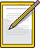Sound pressure and decibels
Music is a mixture of sound pressure at different frequencies.

Sound pressure, or sound pressure level, is the result of the pressure variations in the air achieved by the sound waves. The lowest sound pressure which can be heard by humans is called the hearing threshold, the highest which can be endured is known as the pain threshold. Sound pressure at the pain threshold is a million times greater than that at the hearing threshold.

To avoid having to process numbers over such a wide range, a compressed, logarithmic scale is used based on the decibel unit (dB). Here the lowest sound pressure level has the value of 0 dB (the hearing threshold) while the pain threshold has the value of approximately 120 dB.

If the values are added together on a logarithmic scale, the result differs from that on a linear scale. If two equally strong sound sources are added together, the combined sound pressure level does not double but increases by three decibels.Remember: Sound pressure, or sound pressure level, is the result of the pressure variations in the air achieved by the sound waves The lowest sound pressure which can be heard by humans is called the hearing threshold, the highest which can be endured is known as the pain threshold A compressed, logarithmic scale is used based on the decibel unit (dB) If two equally strong sound sources are added together, the combined sound pressure level does not double but increases by three decibels